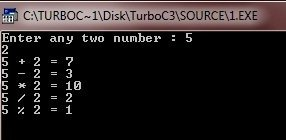# C Operators

C is very rich in built-in operators. There are basically four main classes of operators listed here:

1. arithmetic operator
2. relational operator
3. logical operator
4. bitwise operator

In addition, there are some special types of operators, such as assignment operator, for particular tasks. Now let's discuss about assignment operators.

## C Assignment Operators

You can use the assignment operator within any valid expression. Here is the general form of the assignment operator :

`variable_name = expression;`

where an expression may be as simple as a single constant or as complex as you need.

The target, or left part, of the assignment must be an object, such as a variable, that can receive a value.

### Multiple Assignments

You can also assign many variables the same value by using multiple assignments in a single statement. For instance, here this code fragment assigns a, b, and c, the value 10 :

`a = b = c = 10;`

### Compound Assignments

There is a variation on the assignment statement, called compound assignment, that simplifies the coding of a certain type of assignment operations. For example, this code fragment:

`a = a + 20;`

can be written as

`a += 20;`

Now let's discuss about C Arithmetic Operators.

## C Arithmetic Operators

Here this table lists the C arithmetic operators:

Operator Name
- Subtraction
* Multiplication
/ Division
% Modulus
++ Increment
-- Decrement

The operators, +, -, *, and / works as they do in most other computer languages. You can apply them to almost any built-in data types.

### C Arithmetic Operators Example

Here is a example of C arithmetic operators :

```/* C Arithmetic Operators Program */

#include<stdio.h>
#include<conio.h>
void main()
{
int a, b, res;
clrscr();

printf("Enter any two number : ");
scanf("%d%d", &a, &b);
res = a + b;
printf("%d + %d = %d", a, b, res);
res = a - b;
printf("\n%d - %d = %d", a, b, res);
res = a * b;
printf("\n%d * %d = %d", a, b, res);
res = a / b;
printf("\n%d / %d = %d", a, b, res);
res = a % b;
printf("\n%d %% %d = %d", a, b, res);
getch();
}```

Here is the sample run of the above C program:## C Increment and Decrement Operators

The increment (++) operator simply adds 1 to its operand, and the decrement (--) operator simply subtracts 1 to its operand.

This code fragment

`a = a + 1;`

is same as

`++a;`

and

`a = a - 1;`

is same as

`a--;`

Both the increment and decrement operators may either precede (prefix) or follow (postfix) the operand. For instance,

`a = a + 1;`

can be written as

`++a;`

or

`a++;`

But there is a difference between the prefix and postfix forms when you use the operators in a larger expression. When an increment or decrement operator precedes its operand, the increment or decrement operation is performed before obtaining the value of the operand for use in the expression. If the operator follows its operand, then the value of the operand is obtained before incrementing or decrementing it. For instance,

```a = 20;
b = ++a;```

sets b to 21. On the other hand, if you write the code as

```a = 20;
b = a++;```

b sets to 20. Either way, a is set to 21; the difference is in when it happens.

## C Relational Operators

In the term relational operator, relational refers to the ways these relationships that values can have with one another.

Here this table lists the relational operators.

Operator Name
> Greater than
>= Greater than or equal
< Less than
<= Less than or equal
== Equal
!= Not equal

## C Logical Operators

Here this table lists the C logical operators:

Operator Name
&& AND
|| OR
! NOT

## C Bitwise Operators

Unlike many other languages, C supports a full complement of bitwise operators. Since C was designed to take the place of assembly language for most programming tasks, it needed to be able to support many operations that can be done in assembler, including operations on bits.

Bitwise operation refers to testing, setting, or shifting the actual bits in a byte or word, which corresponds to the standard char and int data types and variants.

Note - You can't use bitwise operations on float, double, long, double, void, or other more complex types.

Here this table lists the bitwise operators that apply to bitwise operations.

Operator Name
& AND
| OR
^ Exclusive OR (XOR)
~ One's complement (NOT)
>> Shift right
<< Shift left

## The ? Operator

C contains a powerful convenient operator that replaces certain statements of the if-then-else form. The ternary operator ? takes the general form :

`Exp1 ? Exp2 : Exp3;`

where Exp1, Exp2, and Exp3 are expressions. Notice the use and placement of the colon.

The ? operator works like this:
Exp1 is evaluated. It it is true, Exp2 is evaluated and becomes the value of the expression. If Exp1 is false, Exp3 is evaluated, and its value becomes the value of the expression. For instance, in

```a = 10;
b = a>9 ? 100 : 200;```

b is assigned the value 100. If a had been less than 9, b would have received the value 200. The same code written using the if-else statement is:

```a = 10;
if(a>9)
b = 100;
else
b = 200;```

## C Operator Precedence

This table lists the C operators with priority or precedence:

Highest
() [] -> .
! ~ ++ -- (type) * & sizeof
* / %
+ -
<< >>
< <= > >=
== !=
&
^
|
&&
||
?:
= += -= *= /= etc.
Lowest

Tools
Calculator